Courses

# Integral of sin5x.cos2x is: a)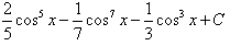b)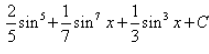c)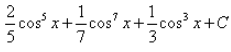d)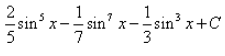Correct answer is 'A'. Can you explain this answer? Related Test: Test: Integration By Substitution

## JEE Question

By Rachit Chauhan · Oct 01, 2018 ·JEE
Shivam Tyagi answered Aug 17, 2018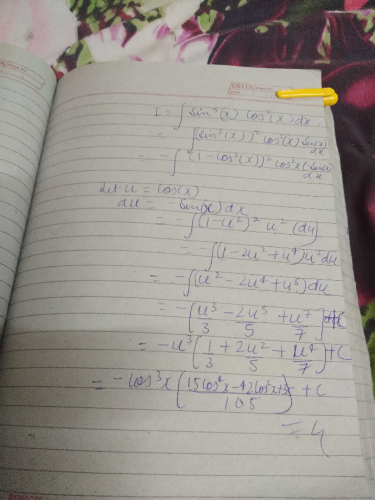Convert it into the form sin x(1-cos^2 x)^2 cos^2 x dx and then take cos x=t

Shashmith Shashmith answered Mar 26, 2020
Convert sinpower4x into cosx then put cosx = t

Suresh Reddy Kopula answered Oct 04, 2018
If you don't mind. Plz give ur num